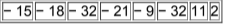×
Get Full Access to Elementary Statistics - 12 Edition - Chapter 3.2 - Problem 17bsc
Get Full Access to Elementary Statistics - 12 Edition - Chapter 3.2 - Problem 17bsc

×

# In Exercises, find the (a)mean, (b) median, | Ch 3.2 - 17BSCISBN: 9780321836960 18

## Solution for problem 17BSC Chapter 3.2

Elementary Statistics | 12th Edition

• Textbook Solutions
• 2901 Step-by-step solutions solved by professors and subject experts
• Get 24/7 help from StudySoup virtual teaching assistantsElementary Statistics | 12th Edition

4 5 1 295 Reviews
31
5
Problem 17BSC

JFK to LAX Flight Delays Listed below are the arrival delay times (in minutes) of randomly selected American Airline flights from New York's JFK airport to Los Angeles (LAX). Negative values correspond to flights that arrived early before the scheduled arrival time, and positive values represent lengths of delays. Based on these very limited results, what do you conclude about the on­time performance of American Airlines? (The data are from the Bureau of Transportation, and more data are listed in Data Set 15 in Appendix B.)Step-by-Step Solution:

Step 1 of 2 :

Given, Listed below are the arrival delay times (in minutes) of randomly selected American Airline flights from New York’s JFK airport to Los Angeles (LAX). Negative values correspond to flights that arrived early before the scheduled arrival time, and positive values represent lengths of delays.Step 2 of 2

##### ISBN: 9780321836960

Since the solution to 17BSC from 3.2 chapter was answered, more than 511 students have viewed the full step-by-step answer. The answer to “?JFK to LAX Flight Delays Listed below are the arrival delay times (in minutes) of randomly selected American Airline flights from New York's JFK airport to Los Angeles (LAX). Negative values correspond to flights that arrived early before the scheduled arrival time, and positive values represent lengths of delays. Based on these very limited results, what do you conclude about the on­time performance of American Airlines? (The data are from the Bureau of Transportation, and more data are listed in Data Set 15 in Appendix B.)” is broken down into a number of easy to follow steps, and 86 words. The full step-by-step solution to problem: 17BSC from chapter: 3.2 was answered by , our top Statistics solution expert on 03/15/17, 10:30PM. This full solution covers the following key subjects: data, given, listed, american, JFK. This expansive textbook survival guide covers 121 chapters, and 3629 solutions. Elementary Statistics was written by and is associated to the ISBN: 9780321836960. This textbook survival guide was created for the textbook: Elementary Statistics, edition: 12.

## Discover and learn what students are asking

Calculus: Early Transcendental Functions : Parametric Equations and Calculus
?In Exercises 1-4, find $$d y / d x$$. $$x=2 e^{\theta}, y=e^{-\theta / 2}$$

Statistics: Informed Decisions Using Data : Properties of the Normal Distribution
?In Problems 25–28, the graph of a normal curve is given. Use the graph to identify the values of ? and ?.

Statistics: Informed Decisions Using Data : Testing the Significance of the Least-Squares Regression Model
?In Problems 5–10, use the results of Problems 7–12, respectively, from Section 4.2 to answer the following questions: (a) What are the estima

Unlock Textbook Solution